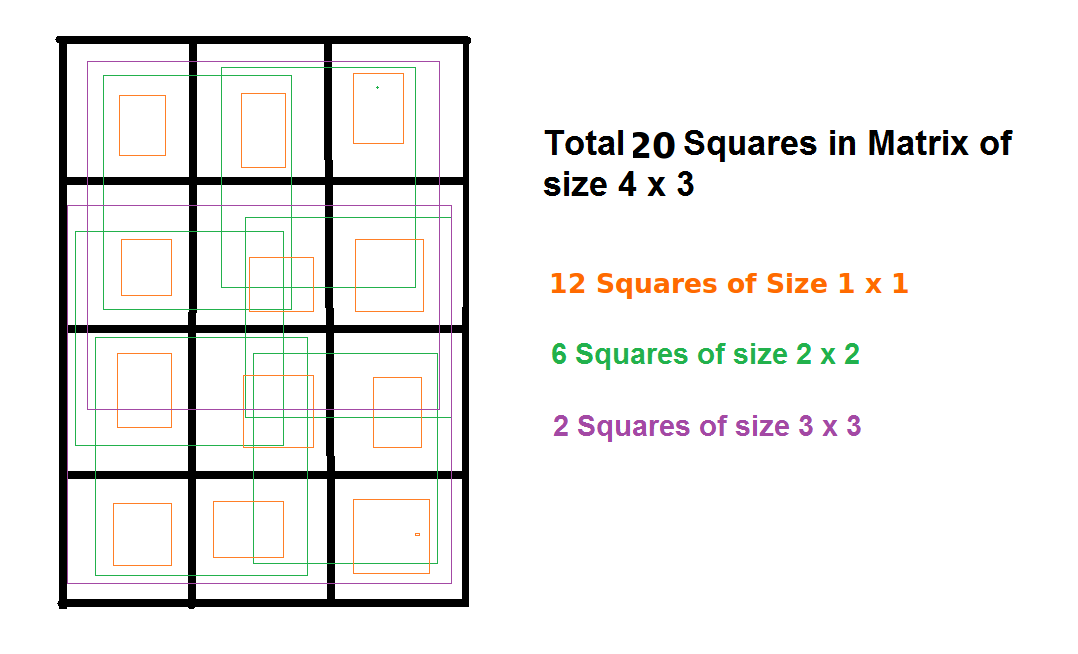GeeksforGeeks App
Open AppBrowser
Continue

# Sum of Area of all possible square inside a rectangle

Given two integers L and B denoting the length and breadth of a rectangle respectively. The task is to calculate the sum of the area of all possible squares that comes into the rectangle.

Examples

```Input: L = 4, B = 3
Output: 54

Input:  L = 2, B = 5
Output: 26```

The idea is to observe the count of number of squares in a rectangle.Now, the number of squares of side 1 will be 12 as there will be two cases one as squares of 1-unit sides along the horizontal(3) and second case as squares of 1-unit sides along the vertical(4). That gives us 3*4 = 12 squares.

When the side is 2 units, one case will be as squares of side of 2 units along only one place horizontally and second case as two places vertically. So the number of squares = 6
So we can deduce that,
Number of squares of size 1*1 will be L*B
Number of squares of size 2*2 will be (L-1)(B-1)
Therefore, the number of squares with sizewill be:

Number of square of size K = (L-K+1)*(B-K+1)

Therefore, area of total number of squares of size K will be:

Area of total number of square of size K = (L-K+1)*(B-K+1)*K*K

Below is the implementation of above idea:

## C++

 `// CPP program to calculate the sum of area``// of all possible squares that comes``// inside the rectangle` `#include ``using` `namespace` `std;` `// Function to calculate the sum of area``// of all possible squares that comes``// inside the rectangle``int` `calculateAreaSum(``int` `l, ``int` `b)``{``    ``int` `size = 1;``    ` `    ``// Square with max size possible``    ``int` `maxSize = min(l,b);``    ` `    ``int` `totalArea = 0;``    ` `    ``for``(``int` `i=1; i <= maxSize; i++)``    ``{  ``        ``// calculate total square of a given size``        ``int` `totalSquares = (l-size+1)*(b-size+1);``        ` `        ``// calculate area of squares of a``        ``// particular size   ``        ``int` `area = totalSquares*size*size;``        ` `        ``// total area   ``        ``totalArea += area;``         ` `        ``// increment size   ``        ``size++;``    ``}``    ` `    ``return` `totalArea;``}` `// Driver Code``int` `main()``{``    ``int` `l = 4, b = 3;``    ` `    ``cout<

## Java

 `// Java program to calculate the``// sum of area of all possible``// squares that comes inside``// the rectangle``class` `GFG``{``// Function to calculate the``// sum of area of all possible``// squares that comes inside``// the rectangle``static` `int` `calculateAreaSum(``int` `l, ``int` `b)``{``    ``int` `size = ``1``;` `    ``// Square with max size possible``    ``int` `maxSize = Math.min(l, b);` `    ``int` `totalArea = ``0``;` `    ``for``(``int` `i = ``1``; i <= maxSize; i++)``    ``{``        ``// calculate total square``        ``// of a given size``        ``int` `totalSquares = (l - size + ``1``) *``                           ``(b - size + ``1``);``    ` `        ``// calculate area of squares``        ``// of a particular size``        ``int` `area = totalSquares *  ``                    ``size * size;``    ` `        ``// total area``        ``totalArea += area;``        ` `        ``// increment size``        ``size++;``    ``}` `    ``return` `totalArea;``}` `// Driver Code``public` `static` `void` `main(String[] args)``{``    ``int` `l = ``4``, b = ``3``;``    ` `    ``System.out.println(calculateAreaSum(l, b));``}``}` `// This code is contributed``// by ChitraNayal`

## Python 3

 `# Python 3 program to calculate``# the sum of area of all possible``# squares that comes inside``# the rectangle` `# Function to calculate the``# sum of area of all possible``# squares that comes inside``# the rectangle``def` `calculateAreaSum(l, b):``    ``size ``=` `1``    ` `    ``# Square with max size possible``    ``maxSize ``=` `min``(l, b)``    ` `    ``totalArea ``=` `0``    ` `    ``for` `i ``in` `range``(``1``, maxSize ``+` `1` `):` `        ``# calculate total square``        ``# of a given size``        ``totalSquares ``=` `((l ``-` `size ``+` `1``) ``*``                        ``(b ``-` `size ``+` `1``))``        ` `        ``# calculate area of squares``        ``# of a particular size``        ``area ``=` `(totalSquares ``*``                ``size ``*` `size)``        ` `        ``# total area``        ``totalArea ``+``=` `area``        ` `        ``# increment size``        ``size ``+``=` `1``        ` `    ``return` `totalArea` `# Driver Code``if` `__name__ ``=``=` `"__main__"``:``    ``l ``=` `4``    ``b ``=` `3``    ` `    ``print``(calculateAreaSum(l,b))` `# This code is contributed``# by ChitraNayal`

## C#

 `// C# program to calculate the``// sum of area of all possible``// squares that comes inside``// the rectangle``using` `System;` `class` `GFG``{``// Function to calculate the``// sum of area of all possible``// squares that comes inside``// the rectangle``static` `int` `calculateAreaSum(``int` `l,``                            ``int` `b)``{``    ``int` `size = 1;` `    ``// Square with max size possible``    ``int` `maxSize = Math.Min(l, b);` `    ``int` `totalArea = 0;` `    ``for``(``int` `i = 1; i <= maxSize; i++)``    ``{``        ``// calculate total square``        ``// of a given size``        ``int` `totalSquares = (l - size + 1) *``                           ``(b - size + 1);``    ` `        ``// calculate area of squares``        ``// of a particular size``        ``int` `area = totalSquares *``                   ``size * size;``    ` `        ``// total area``        ``totalArea += area;``        ` `        ``// increment size``        ``size++;``    ``}``    ``return` `totalArea;``}` `// Driver Code``public` `static` `void` `Main()``{``    ``int` `l = 4, b = 3;``    ` `    ``Console.Write(calculateAreaSum(l,b));``}``}` `// This code is contributed``// by ChitraNayal`

## PHP

 ``

## Javascript

 ``

Output

`54`

Complexity Analysis:

• Time complexity: O(min(l,b))
• Auxiliary complexity: O(1)

My Personal Notes arrow_drop_up